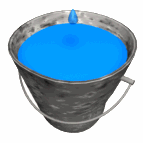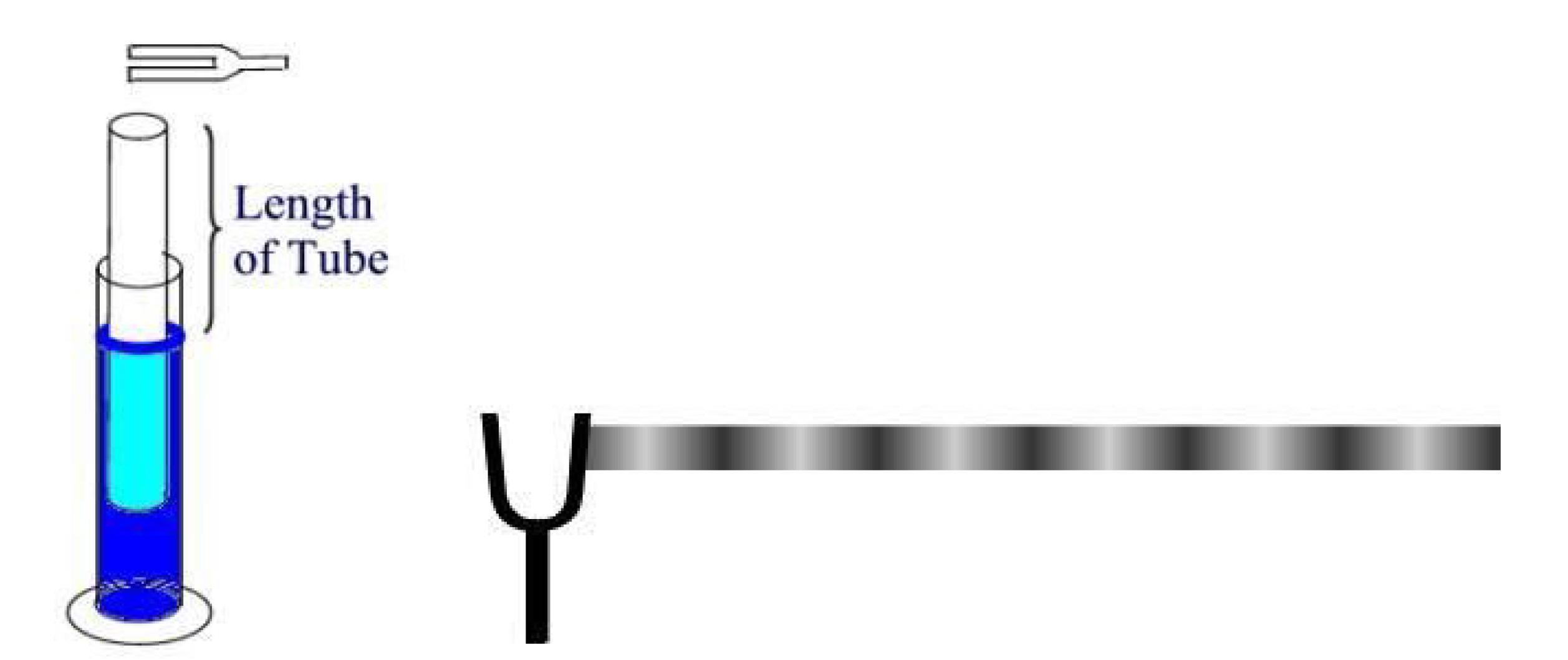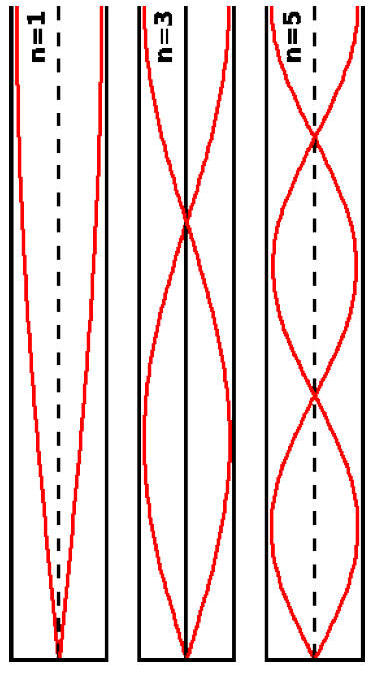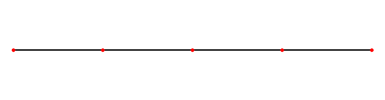Homeshowmethephysics.com

II. Standing Wave

- One end open, other end closedA. This standing wave is caused by reflection

B. There is always a NODE on the closed end and an ANTINODE on the open end

1. 1st Harmonic (Fundamental) (N.A.)

N.A. = (Node - Antinode)

L = Standing Wave LengthL = 1/4 l  l = 4L L = 3/4 l  l = (4/3)L L = 5/4 l  l = (4/5)L

2. 3rd Harmonic (NA NA)

L = Standing Wave LengthL = 1/4 l  l = 4L L = 3/4 l  l = (4/3)L L = 5/4 l  l = (4/5)L

3. 5th Harmonic (NA NA NA)

L = Standing Wave LengthL = 1/4 l  l = 4L L = 3/4 l  l = (4/3)L L = 5/4 l  l = (4/5)L

4. 7th Harmonic (NA NA NA NA)

L (Standing Wave Length) = 7/4 l

l = (4/7)L

L= [n/4]l

n = 1, 3, 5, 7 .....

since v = fl

then

fn = nv/4L

n = 1,3,5,7 .....

If fundamental is 20 Hz

Then a standing wave can be produced at
60 Hz, 100 Hz, 140 Hz

showmethephysics.com

D. Open at Both EndsL = 2/4 l  l = 2L L = 4/4 l  l = L L = 6/4 l  l = (2/3)L L = 8/4 l  l = (1/2)L

1/2, 2/2, 3/2, 4/2

L = n(1/2)λ

2L/n = λ

since v = fl

since v/l = f

then

fn = nv/2L

n = 1,2,3,4 .....

Beats - Rhythmic interference between two semi identical frequency sources

Beat Frequency = |f1 - f2|ResonanceEnrichmentBerkeley Univ.Water Drop in Zero gStanding Waves
HyperPhysics (offsite
)